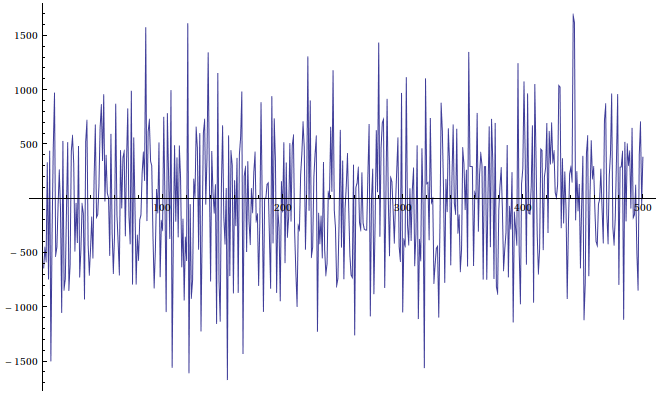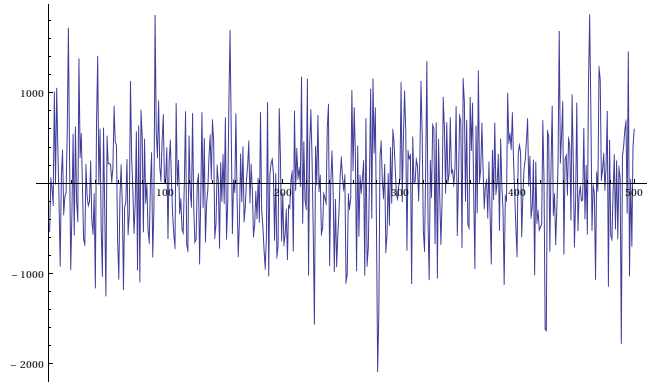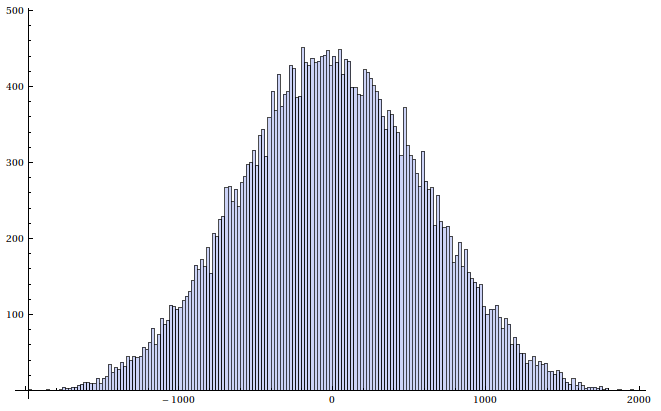Date

The other day I was coding an FPGA design that needed a source of normally distributed random numbers. Uniformly distributed random numbers are fairly easy and cheap to generate with a linear feedback shift register, but generating normally distributed numbers is a harder problem. There are several ways to generate a normally distributed number using one or more uniformly distributed, some are more accurate than others. For my purposes the numbers don't need to exactly follow the normal distribution,, it's enough if it seems like for a normal human that the numbers are from the normal distribution.

So I went for the easiest and cheapest implementation and use the central limit theorem, which says that summing sufficiently many independent random numbers result will be approximately normally distributed. I went for the sum of four uniformly distributed random numbers generated by a linear feedback shift register. To avoid obvious period in the output I decided to have a rather large 31-bit registers. 31-bits because it's big enough and it needs only two taps when a 32-bit register would need four.

Code for the linear feedback shift registers:

 ``` 1 2 3 4 5 6 7 8 9 10 11 12 13 14 15 16 17 18 19 20 21 22 23 24 25 26 27 28 29 30 31 32 33``` ``````library IEEE; use IEEE.STD_LOGIC_1164.ALL; entity random_uniform is generic ( SEED : STD_LOGIC_VECTOR(30 downto 0):= (others => '0'); OUT_WIDTH : integer := 11); Port ( clk : in STD_LOGIC; random : out STD_LOGIC_VECTOR (OUT_WIDTH-1 downto 0); reset : in STD_LOGIC); end random_uniform; architecture Behavioral of random_uniform is signal rand : std_logic_vector(30 downto 0) := SEED; signal feedback : std_logic; begin feedback <= not((rand(0) xor rand(3))); process(clk,reset) begin if reset = '1' then rand <= SEED; elsif rising_edge(clk) then rand <= feedback&rand(30 downto 1); end if; end process; random <= rand(OUT_WIDTH-1 downto 0); end Behavioral; ``````

Implementation of the linear feedback shift registers is very straight forward. Seed value and output width can be set with generics making it also useful in many other places.

Summing four of these uniform random numbers should generate number that is almost normally distributed. Code for the normally distributed pseudorandom generator is below, it outputs 12-bit signed numbers every three clock cycles, but it should be easy to modify it to work in one clock cycle just by adding more adders.

 ``` 1 2 3 4 5 6 7 8 9 10 11 12 13 14 15 16 17 18 19 20 21 22 23 24 25 26 27 28 29 30 31 32 33 34 35 36 37 38 39 40 41 42 43 44 45 46 47 48 49 50 51 52 53 54 55 56 57 58 59 60 61 62 63 64 65 66 67 68 69 70 71 72 73 74 75 76 77 78 79 80 81 82 83 84 85 86 87 88 89 90 91 92 93 94 95 96 97 98 99 100 101 102 103 104 105 106 107 108 109 110 111 112 113 114 115 116 117 118 119 120 121 122 123 124 125 126 127 128 129 130 131 132 133 134 135``` ``````library IEEE; use IEEE.STD_LOGIC_1164.ALL; use IEEE.NUMERIC_STD.ALL; entity random_gaussian is Port ( clk : in STD_LOGIC; reset : in STD_LOGIC; random : out STD_LOGIC_VECTOR (11 downto 0)); end random_gaussian; architecture Behavioral of random_gaussian is component random_uniform is generic ( SEED : STD_LOGIC_VECTOR(30 downto 0); OUT_WIDTH : integer); port( clk : in STD_LOGIC; random : out STD_LOGIC_VECTOR (OUT_WIDTH-1 downto 0); reset : in STD_LOGIC); end component; component adder_signed is Port ( clk : in STD_LOGIC; a : in STD_LOGIC_VECTOR (11 downto 0); b : in STD_LOGIC_VECTOR (11 downto 0); r : out STD_LOGIC_VECTOR (11 downto 0)); end component; signal uniform1 : std_logic_vector(9 downto 0); signal uniform2 : std_logic_vector(9 downto 0); signal uniform3 : std_logic_vector(9 downto 0); signal uniform4 : std_logic_vector(9 downto 0); signal adder_a : std_logic_vector(11 downto 0); signal adder_b : std_logic_vector(11 downto 0); signal adder_r : std_logic_vector(11 downto 0); type statetype is (s0, s1, s2); signal state, next_state: statetype := s0; begin unif1: random_uniform generic map (SEED => std_logic_vector(to_unsigned(697757461,31)), OUT_WIDTH => 10) port map( clk => clk, random => uniform1, reset => reset ); unif2: random_uniform generic map (SEED => std_logic_vector(to_unsigned(1885540239,31)), OUT_WIDTH => 10) port map( clk => clk, random => uniform2, reset => reset ); unif3: random_uniform generic map (SEED => std_logic_vector(to_unsigned(1505946904,31)), OUT_WIDTH => 10) port map( clk => clk, random => uniform3, reset => reset ); unif4: random_uniform generic map (SEED => std_logic_vector(to_unsigned(2693445,31)), OUT_WIDTH => 10) port map( clk => clk, random => uniform4, reset => reset ); adder1 : adder_signed PORT MAP ( a => adder_a, b => adder_b, clk => clk, r => adder_r ); process(clk, reset) begin if rising_edge(clk) then if reset = '1' then state <= s0; random <= (others => '0'); else if state = s0 then random <= adder_r; end if; state <= next_state; end if; end if; end process; process(clk,state,uniform1,uniform2,uniform3,uniform4,adder_r) begin case state is when s0 => -- Sign extend adder_a <= uniform1(9)&uniform1(9)&uniform1(9)&uniform1(8 downto 0); adder_b <= uniform2(9)&uniform2(9)&uniform2(9)&uniform2(8 downto 0); next_state <= s1; when s1 => adder_a <= adder_r; adder_b <= uniform3(9)&uniform3(9)&uniform3(9)&uniform3(8 downto 0); next_state <= s2; when s2 => adder_a <= adder_r; adder_b <= uniform4(9)&uniform4(9)&uniform4(9)&uniform4(8 downto 0); next_state <= s0; end case; end process; end Behavioral; ``````

And the code of the adder for adding two 12-bit signed numbers, it has a latency of one cycle:

 ``` 1 2 3 4 5 6 7 8 9 10 11 12 13 14 15 16 17 18 19 20 21 22 23 24 25 26 27 28 29``` ``````library IEEE; use IEEE.STD_LOGIC_1164.ALL; use IEEE.NUMERIC_STD.ALL; entity adder_signed is Port ( clk : in STD_LOGIC; a : in STD_LOGIC_VECTOR (11 downto 0); b : in STD_LOGIC_VECTOR (11 downto 0); r : out STD_LOGIC_VECTOR (11 downto 0)); end adder_signed; architecture Behavioral of adder_signed is signal r_next : std_logic_vector(11 downto 0) := (others => '0'); begin process(clk) begin if rising_edge(clk) then r <= r_next; r_next <= std_logic_vector(signed(a)+signed(b)); end if; end process; end Behavioral; ``````

I wrote a testbench to write output values to a file and a mathematica notebook to test how close the numbers are to the normal distribution. Histograms were generated with "Histogram[x, 200]" command and plots with "ListPlot[x, Joined->True]", where "x" is a list of values.500 output values of the generator.Same amount of samples from the normal distribution.

You can see that the approximation is pretty close to the normal distribution. It's maybe a little bit fatter, but it wouldn't be noticeable without comparing it to a true normal distribution.Histogram of the generated numbers. Does it look like a normal distribution?Histogram of the same amount of random numbers generated from the normal distribution. Notice the different values on the axes.

As you can see from the histograms the approximation is a little bit flatter, but it comes amazingly close. Just by looking at the histogram without a normal distribution to compare it to, I would say that it is a normal distribution and that's the only thing that was important.

Appendix 1.

VHDL test bench for writing the output values into a file:

 ``` 1 2 3 4 5 6 7 8 9 10 11 12 13 14 15 16 17 18 19 20 21 22 23 24 25 26 27 28 29 30 31 32 33 34 35 36 37 38 39 40 41 42 43 44 45 46 47 48 49 50 51 52 53 54 55 56 57 58 59 60 61 62 63 64 65 66 67 68 69 70 71 72 73 74``` ``````LIBRARY ieee; USE ieee.std_logic_1164.ALL; use std.textio.all; use ieee.std_logic_textio.all; USE ieee.numeric_std.ALL; ENTITY random_gaussian_test_bench IS END random_gaussian_test_bench; ARCHITECTURE behavior OF random_gaussian_test_bench IS FILE test_out_data: TEXT open WRITE_MODE is "output/gaussian.txt"; -- Component Declaration for the Unit Under Test (UUT) COMPONENT random_gaussian PORT( clk : IN std_logic; reset : IN std_logic; random : OUT std_logic_vector(11 downto 0) ); END COMPONENT; --Inputs signal clk : std_logic := '0'; signal reset : std_logic := '0'; --Outputs signal random : std_logic_vector(11 downto 0); -- Clock period definitions constant clk_period : time := 10 ns; BEGIN -- Instantiate the Unit Under Test (UUT) uut: random_gaussian PORT MAP ( clk => clk, reset => reset, random => random ); -- Clock process definitions clk_process :process begin clk <= '0'; wait for clk_period/2; clk <= '1'; wait for clk_period/2; end process; -- Stimulus process stim_proc: process variable L1 : LINE; variable I : integer; begin reset <= '1'; wait for clk_period*20; reset <= '0'; wait for clk_period*30; for I in 0 to 1000000 loop wait for clk_period*3; write(L1, to_integer(signed(random))); writeline(test_out_data, L1); end loop; wait; end process; END; ``````